Chapter 18. Chemical Thermodynamics

# Spontaneity: Free Energy and Temperature

Jessie A. Key

Learning Objectives

• To gain an understanding of the relationship between spontaneity, free energy, and temperature.
• To be able to calculate the temperature at which a process is at equilibrium under standard conditions.

In the Gibbs free energy change equation, the only part we as scientists can control is the temperature. We have seen how we can calculate the standard change in Gibbs free energy, ΔG°, but not all reactions we are interested in occur at exactly 298 K. The temperature plays an important role in determining the Gibbs free energy and spontaneity of a reaction.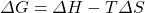If we examine the Gibbs free energy change equation, we can cluster the components to create two general terms, an enthalpy term, ΔH, and an entropy term, –TΔS. Depending on the sign and magnitude of each, the sum of these terms determines the sign of ΔG and therefore the spontaneity (Table 18.2 “Spontaneity and the Signs of Enthalpy and Entropy Terms”).

Table 18.2 Spontaneity and the Signs of Enthalpy and Entropy Terms
ΔH ΔS TΔS ΔG Spontaneity
+ + + Nonspontaneous
+ Spontaneous
+ + or −
• Low temp: Spontaneous
• High temp: Nonspontaneous
+ + + or −
• Low temp: Nonspontaneous
• High temp: Spontaneous

Since all temperature values are positive in the Kelvin scale, the temperature affects the magnitude of the entropy term. As shown in Table 18.2 “Spontaneity and the Signs of Enthalpy and Entropy Terms,” the temperature can be the deciding factor in spontaneity when the enthalpy and entropy terms have opposite signs. If ΔH is negative, and –TΔS positive, the reaction will be spontaneous at low temperatures (decreasing the magnitude of the entropy term). If ΔH is positive, and –TΔS negative, the reaction will be spontaneous at high temperatures (increasing the magnitude of the entropy term).

Sometimes it can be helpful to determine the temperature when ΔG° = 0 and the process is at equilibrium. Knowing this value, we can adjust the temperature to drive the process to spontaneity or alternatively to prevent the process from occurring spontaneously. Remember that, at equilibrium: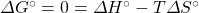We can rearrange and solve for the temperature T: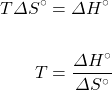Example 18.6

Using the appendix table of standard thermodynamic quantities, determine the temperature at which the following process is at equilibrium: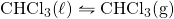How does the value you calculated compare to the boiling point of chloroform given in the literature?

Solution

At equilibrium:We must estimate ΔH° and S° from their enthalpies of formation and standard molar entropies, respectively.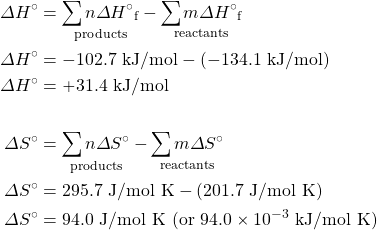Now we can use these values to solve for the temperature: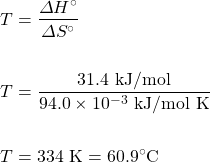The literature boiling point of chloroform is 61.2°C. The value we have calculated is very close but slightly lower due to the assumption that ΔH° and S° do not change with temperature when we estimate the ΔH° and S° from their enthalpies of formation and standard molar entropies.

Key Takeaways

• The temperature can be the deciding factor in spontaneity when the enthalpy and entropy terms have opposite signs:
• If ΔH is negative, and –TΔpositive, the reaction will be spontaneous at low temperatures (decreasing the magnitude of the entropy term).
• If ΔH is positive, and –TΔnegative, the reaction will be spontaneous at high temperatures (increasing the magnitude of the entropy term).